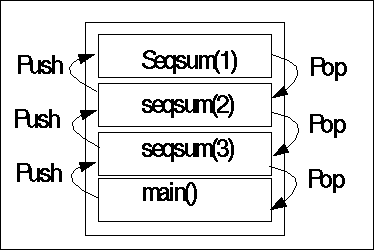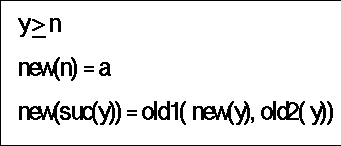## I-ALGO SS03 - ALGORITHMS AND DATASTRUCTURES - Lecture with Exercices Recursion

```                         Attention : This text is unfinished due to some kind of influenza...
The written text does not fully represent the oral lecture !!!
```

AUTHOR: Gerd Döben-Henisch
DATE OF FIRST GENERATION: March-18, 2003
DATE OF LAST CHANGE: March-26, 2003
EMAIL: Gerd Döben-Henisch

#### 1. Introduction

In this lecture we will present the concept of Recursion.

START

#### 2. Recursion: Introductory Example

In an informal way it is often said that a recursive function is a function which makes directly or indirectly a call to itself, like in the following simple example seqsum(), where the function seqsum() computes the sum of an initial sequence of the natural numbers, i.e.

seqsum(n) = SUM(i=1,n) i

seqsum(4) = 1 + 2 + 3 + 4 = 10

```
//---------------------------
//
// seqsum.cpp
//
// example of simple recursion
// computes the sum of a sequence
//
// compile: g++ -o seqsum seqsum.cpp
// usage: seqsum
//
//--------------------------------------

#include <iostream>
using namespace std;

int main( void )
{
extern int seqsum(int);

int n;
cout << "Enter maximal element for summation: " << endl;
cin >> n;
cout << "Result = " << seqsum(n) << endl;

return 0;
}

int seqsum(int n){

if (n == 1) return 1;
else return seqsum(n-1)+n;

}

```
```gerd@turing:~/public_html/I-ALGO/I-ALGO-EX/EX2> seqsum
Enter maximal element for summation:
4
Result = 10

```

In this function seqsum() calls itself but with a different argument. What looks at a first glance a bit like 'magic' reveals itself as a simple and straightforward mechanism.

Behind the 'surface' of the programming language expressions will the compiler translate this code in a machine readable code and when the automaton is processing functions it will use a stack to store the called functions before their evaluations.Function Stack

But this informal characterization of recursive functions as functions which call themselves is too simple as to give a full account of what recursive functions are. A more theoretical explanation follows in the next paragraph.

START

#### 3. Recursion: General Scheme

A slightly more general schema for recursive functions is given in the diagram below.Recursive Function Schema

This schema consists of two parts: in part one (new(n)=a)a function 'new' is declared for a base-case with a fixed value a.In part two (new(suc(y)) ) old1(new(y),old2(y)) we find the more complex schema connecting a new value suc(y) of new with the old value y of new. This is done with the help of some already know functions 'old1' and 'old2'.

Lets look to some examples:

/* Sum */
sm(0,x) = x
sm(suc(y),x) = suc(sm(y,x))

sm(2,3)
suc(sm(1,3))
suc(suc(sm(0,3)))
suc(suc(3))
suc(4)
5

/* Sequential Sum */
ss(1) = 1
ss(suc(y)) = sm(ss(y),suc(y))

ss(3)
sm(ss(2),suc(2)))
sm(sm(ss(1),suc(1)),suc(2)))
sm(sm(1,2),3))
sm(suc(sm(0,2)),3))
sm(suc(2),3))
sm(3,3)
...
6

/* Product */
prod(0,x) = 0
prod(suc(y),x) = sm(prod(y,x),x)

prod(2,2)
sm(prod(1,2),2)
sm(sm(prod(0,2),2),2)
sm(sm(0,2),2)
sm(2,2)
...
4

/* Factorial */
n < 1 fac(n) = 1
fac(suc(y)) = prod(fac(y),suc(y))

fac(3)
prod(fac(2),suc(2))
prod(prod(fac(1),suc(1)),suc(2))
prod(prod(1,2),3)
prod(sm(prod(0,2),2),3)
prod(sm(0,2),3)
prod(2,3)
...
6

/* Fibonacci */
n < 0
fib(n) = 1
fib(suc(y)) = sm(fib(y),fib(vg(y)))

1, 2, 3, 5, 8, 13, ...

position 0: 1
position 1: 2
position 2: 3
...

fib(3)
sm(fib(2),fib(vg(2)))
sm(sm(fib(1),fib(vg(1))),sm(fib(0),fib(vg(0))) )
sm(sm(sm(fib(0),fib(vg(0))),1),sm(1,1) )
sm(sm(sm(1,1),1),sm(1,1) )
sm(sm(2,1),2 )
sm(3,2 )
5

START

#### 3. Recursion Theory

There was an explanation about the Induction principle and why and how this principle can give the theoretical basis for recursive definitions.

START

#### 4. Fibonacci Counterexample

With the recursive definition of the fibonacci numbers an example was given, which has been analyzed and which showed that the time capacities needed for such an algorithm can be in the order of O(N2). Thus recursion has to be used with 'care'.

START

START

START

START